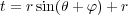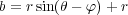## Appendix EPerl Scripts Used

Out of sheer boredom, I provided the Perl script used to make the diagram seen in figure A.6. It is seen in figure E.1.

 #!/usr/bin/perl -w  #  # \$Id: perl.tex,v 1.5 2003/07/13 14:52:58 gleepy Exp \$  # Generates the coordinates necessary to draw the blowin doors used  # in blowin.tex.  #    \$pi = atan2(1, 1) * 4;  \$d = 8.0 * \$pi / 180.0;  # 8 degrees in radians, half the door angle  \$step = 20.0 * \$pi / 180.0;    for (\$i = -4; \$i <= 4; ++\$i) {       \$th = \$i * \$step;  # 20 degree spacing in radians     \$bottom = sin(\$th - \$d) * 60.0 + 60.0;     \$top = sin(\$th + \$d) * 60.0 + 60.0;       printf("\\put(195,%9.4f){\\line(1,0){10.0000}}\n", \$bottom);     printf("\\put(195,%9.4f){\\line(1,0){10.0000}}\n", \$top);     printf("\\put(195,%9.4f){\\line(0,1){%7.4f}}   %% blow-in door %2d\n",      \$bottom, \$top - \$bottom, \$i);     printf("\\put(205,%9.4f){\\line(0,1){%7.4f}}\n",      \$bottom, \$top - \$bottom);     print "\n";  }

 Figure E.1: Perl script used to make the blow-in doors

The key to making the diagram work is to remember that I am representing a circle in what is called an orthographic projection. I use the basic formulas(E.1)

and(E.2)

where r is the radius of the shape, such that it is drawn with a length of 2r, t is the position of the top of the line, b is the position of the bottom of the line, θ is the angle which the door is centered on, and φ is the angular size of the door. From that, the expression t - b is the length of the two vertical lines for each door.